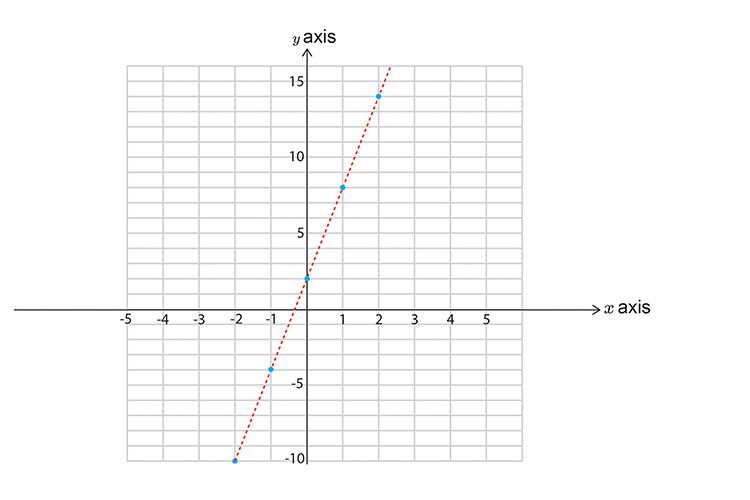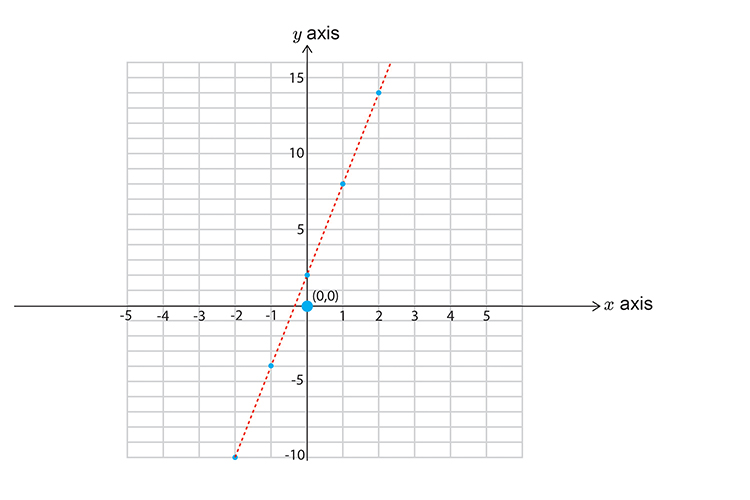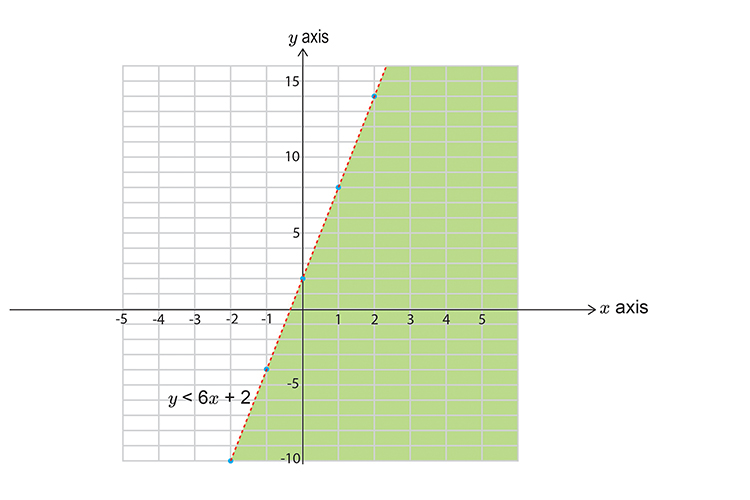# Inequalities and graphs example 3

Solve the inequality

6x-y>\-2

Subtract 6x from both sides to get y on its own.

6x-6x-y>\-2-6x

-y>\-2-6x

Divide both sides by -1  to get a positive. Don’t forget to FLIP the sign.

(-y)/\-1<-(2x)/-1-(6x)/-1

y<\2+6x

or y<\6x+2

First let's calculate points on the graph

x=-2       then   y<\6(-2)+2=-12+2=-10

x=-1       then   y<\6(-1)+2=-6+2=-4

x=0             then   y<\6(0)+2=0+2=2

x=1             then   y<\6times1+2=6+2=8

x=2             then   y<\6times2+2=12+2=14

Now draw a graph and mark on the above points. Don’t forget to use a DOTTED line.Now pick a point NOT on the lineChoose   x=0   y=0

y<\6x+2

0<\6(0)+2

0<\2

This reads zero is less than two.

This does satisfy the inequality, SHADE IT.